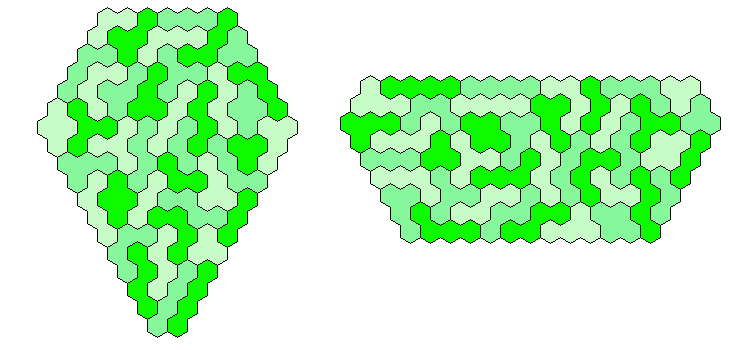## Three Sets of Two-sided Colored Trihexes and Tetrahexes

There are 3*3=9 trihexes and 7*3=21 tetrahexes with a total area of 27+84=111 hexagons. A semi regular hexagon of size 3-11-3-11-3-11 can be constructed.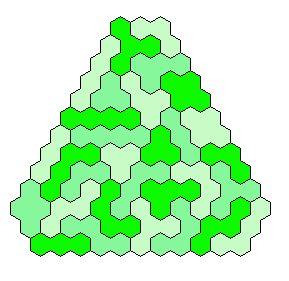Because 37*3=111 and a size 4 hexagon has 37 single hexagons I tried to make three of them. Threefold copies of other shapes are also possible.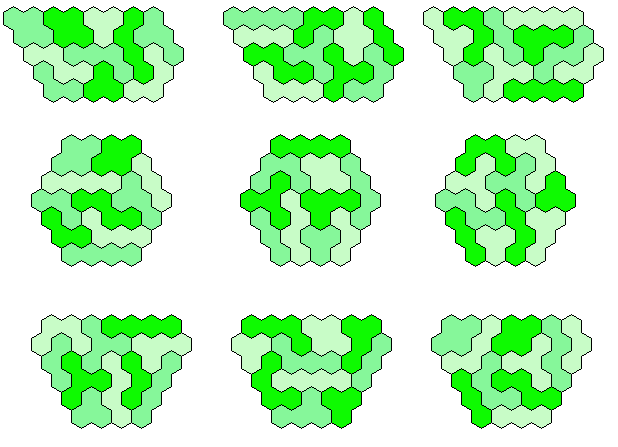Since 37 and 3 are primes only one parallelogram can be made.Solving a(a+1) - b(b+1), 0 < b, b < a leads to two trapezia.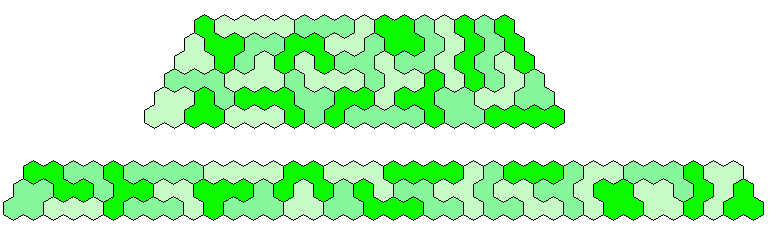I found two pentagonal symmetric patterns.Three hexagons with one axis of symmetry are shown.## Three Sets of One-sided Colored Trihexes and Tetrahexes

We have 3*3=9 trihexes and 10*3=30 tetrahexes with a total area of 27+120=147 hexagons and we can get a semi regular hexagon with triangle symmetry.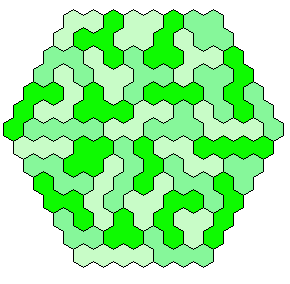A kind of similar hole figure is a triangle of size 17 with a triangular hole of size 3.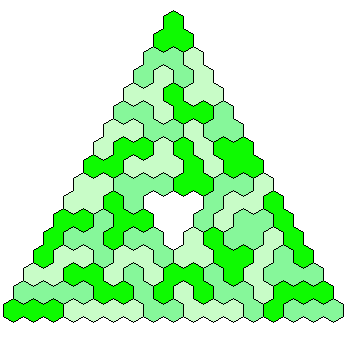Since 147=49*3 and 49 is a square, three congruent rhombs can be made.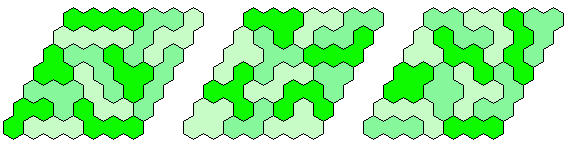147=3*7*7. Solution for both parallelograms 3x49 and 7x21 are given.There are four trapezia with height > 2 and I covered all of them with the pieces.There is also a pentagonal symmetric pattern with an area of 147.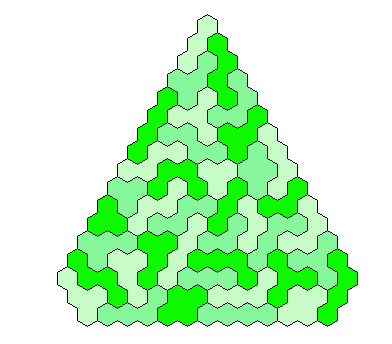At last two solutions for hexagonal, symmetric shapes are added.